Course Content
Class 7th Science
0/36
Class 7th Math
0/71
Class 7 Social Science Geography
0/20
Class 7 Social Science History
0/20
Class 7 Social Science Civics
0/19
Class 7 English Honeycomb
0/20
Class 7 English Honeycomb Poem
0/18
Class 7 English An Alien Hand Supplementary
0/20
Online Class For 7th Standard Students (CBSE)

### NCERT Solutions For Class 7 Maths Chapter 2 – Fractions and Decimals

Exercise 2.1 Page: 31

1. Solve:

(i) 2 – (3/5)

Solution:-

For subtraction of two unlike fractions, first change them to the like fractions.

LCM of 1, 5 = 5

Now, let us change each of the given fraction into an equivalent fraction having 5 as the denominator.

= [(2/1) × (5/5)] = (10/5)

= [(3/5) × (1/1)] = (3/5)

Now,

= (10/5) – (3/5)

= [(10 – 3)/5]

= (7/5)

(ii) 4 + (7/8)

Solution:-

For addition of two unlike fractions, first change them to the like fractions.

LCM of 1, 8 = 8

Now, let us change each of the given fraction into an equivalent fraction having 8 as the denominator.

= [(4/1) × (8/8)] = (32/8)

= [(7/8) × (1/1)] = (7/8)

Now,

= (32/8) + (7/8)

= [(32 + 7)/8]

= (39/8)

=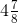(iii) (3/5) + (2/7)

Solution:-

For addition of two unlike fractions, first change them to the like fractions.

LCM of 5, 7 = 35

Now, let us change each of the given fraction into an equivalent fraction having 35 as the denominator.

= [(3/5) × (7/7)] = (21/35)

= [(2/7) × (5/5)] = (10/35)

Now,

= (21/35) + (10/35)

= [(21 + 10)/35]

= (31/35)

(iv) (9/11) – (4/15)

Solution:-

For subtraction of two unlike fractions, first change them to the like fractions.

LCM of 11, 15 = 165

Now, let us change each of the given fraction into an equivalent fraction having 165 as the denominator.

= [(9/11) × (15/15)] = (135/165)

= [(4/15) × (11/11)] = (44/165)

Now,

= (135/165) – (44/165)

= [(135 – 44)/165]

= (91/165)

(v) (7/10) + (2/5) + (3/2)

Solution:-

For addition of two unlike fractions, first change them to the like fractions.

LCM of 10, 5, 2 = 10

Now, let us change each of the given fraction into an equivalent fraction having 35 as the denominator.

= [(7/10) × (1/1)] = (7/10)

= [(2/5) × (2/2)] = (4/10)

= [(3/2) × (5/5)] = (15/10)

Now,

= (7/10) + (4/10) + (15/10)

= [(7 + 4 + 15)/10]

= (26/10)

= (13/5)

=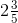(vi)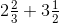Solution:-

First convert mixed fraction into improper fraction,

=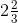= 8/3

= 3 ½ = 7/2

For addition of two unlike fractions, first change them to the like fractions.

LCM of 3, 2 = 6

Now, let us change each of the given fraction into an equivalent fraction having 6 as the denominator.

= [(8/3) × (2/2)] = (16/6)

= [(7/2) × (3/3)] = (21/6)

Now,

= (16/6) + (21/6)

= [(16 + 21)/6]

= (37/6)

=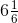(vii) [latex]8frac{1}{2}-3frac{5}{8}[/latex]

Solution:-

First convert mixed fraction into improper fraction,

= 8 ½ = 17/2

=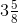= 29/8

For Subtraction of two unlike fractions, first change them to the like fractions.

LCM of 2, 8 = 8

Now, let us change each of the given fraction into an equivalent fraction having 35 as the denominator.

= [(17/2) × (4/4)] = (68/8)

= [(29/8) × (1/1)] = (29/8)

Now,

= (68/8) – (29/8)

= [(68 – 29)/8]

= (39/8)

=2. Arrange the following in descending order:

(i) 2/9, 2/3, 8/21

Solution:-

LCM of 9, 3, 21 = 63

Now, let us change each of the given fraction into an equivalent fraction having 63 as the denominator.

[(2/9) × (7/7)] = (14/63)

[(2/3) × (21/21)] = (42/63)

[(8/21) × (3/3)] = (24/63)

Clearly,

(42/63) > (24/63) > (14/63)

Hence,

(2/3) > (8/21) > (2/9)

Hence, the given fractions in descending order are (2/3), (8/21), (2/9)

(ii) 1/5, 3/7, 7/10

Solution:-

LCM of 5, 7, 10 = 70

Now, let us change each of the given fraction into an equivalent fraction having 70 as the denominator.

[(1/5) × (14/14)] = (14/70)

[(3/7) × (10/10)] = (30/70)

[(7/10) × (7/7)] = (49/70)

Clearly,

(49/70) > (30/70) > (14/70)

Hence,

(7/10) > (3/7) > (1/5)

Hence, the given fractions in descending order are (7/10), (3/7), (1/5)

3. In a “magic square”, the sum of the numbers in each row, in each column and along the diagonals is the same. Is this a magic square?

 4/11 9/11 2/11 3/11 5/11 7/11 8/11 1/11 6/11

Solution:-

Sum along the first row = (4/11) + (9/11) + (2/11) = (15/11)

Sum along the second row = (3/11) + (5/11) + (7/11) = (15/11)

Sum along the third row = (8/11) + (1/11) + (6/11) = (15/11)

Sum along the first column = (4/11) + (3/11) + (8/11) = (15/11)

Sum along the second column = (9/11) + (5/11) + (1/11) = (15/11)

Sum along the third column = (2/11) + (7/11) + (6/11) = (15/11)

Sum along the first diagonal = (4/11) + (5/11) + (6/11) = (15/11)

Sum along the second diagonal = (2/11) + (5/11) + (8/11) = (15/11)

Yes. The sum of the numbers in each row, in each column and along the diagonals is the same, so it is a magic square.

4. A rectangular sheet of paper is 12 ½ cm long and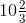cm wide. Find its perimeter.

Solution:-

From the question, it is given that,

Length = 12 ½ cm = 25/2 cmcm = 32/3 cm

We know that,

Perimeter of the rectangle = 2 × (length + breadth)

= 2 × [(25/2) + (32/3)]

= 2 × {[(25 × 3) + (32 × 2)]/6}

= 2 × [(75 + 64)/6]

= 2 × [139/6]

= 139/3 cm

Hence, the perimeter of the sheet of paper is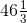cm

5. Find the perimeters of (i) Triangle ABE (ii) the rectangle BCDE in this figure. Whose perimeter is greater?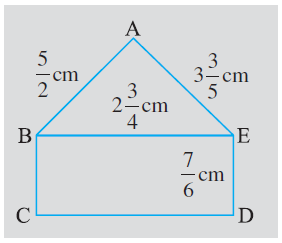Solution:-

From the fig,

AB = (5/2) cm

AE =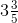= 18/5 cm

BE =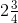= 11/4 cm

ED = 7/6 cm

(i) We know that,

Perimeter of the triangle = Sum of all sides

Then,

Perimeter of triangle ABE = AB + BE + EA

= (5/2) + (11/4) + (18/5)

The LCM of 2, 4, 5 = 20

Now, let us change each of the given fraction into an equivalent fraction having 20 as the denominator.

= {[(5/2) × (10/10)] + [(11/4) × (5/5)] + [(18/5) × (4/4)]}

= (50/20) + (55/20) + (72/20)

= (50 + 55 + 72)/20

= 177/20

=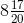cm

(ii) Now, we have to find the perimeter of the rectangle,

We know that,

Perimeter of the rectangle = 2 × (length + breadth)

Then,

Perimeter of rectangle BCDE = 2 × (BE + ED)

= 2 × [(11/4) + (7/6)]

The LCM of 4, 6 = 12

Now, let us change each of the given fraction into an equivalent fraction having 20 as the denominator

= 2 × {[(11/4) × (3/3)] + [(7/6) × (2/2)]}

= 2 × [(33/12) + (14/12)]

= 2 × [(33 + 14)/12]

= 2 × (47/12)

= 47/6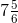Finally, we have find which one is having greater perimeter.

Perimeter of triangle ABE = (177/20)

Perimeter of rectangle BCDE = (47/6)

The two perimeters are in the form of unlike fraction.

Changing perimeters into like fractions we have,

(177/20) = (177/20) × (3/3) = 531/60

(43/6) = (43/6) × (10/10) = 430/60

Clearly, (531/60) > (430/60)

Hence, (177/20) > (43/6)

∴ Perimeter of Triangle ABE > Perimeter of Rectangle (BCDE)

6. Salil wants to put a picture in a frame. The picture is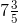cm wide. To fit in the frame the picture cannot be more than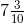cm wide. How much should the picture be trimmed?

Solution:-

From the question, it is given that,

Picture having a width of == 38/5 cm

Frame having a width of == 73/10 cm

∴The picture should be trimmed by = [(38/5) – (73/10)]

The LCM of 5, 10 = 10

Now, let us change each of the given fraction into an equivalent fraction having 10 as the denominator.

= [(38/5) × (2/2)] – [(73/10) × (1/1)]

= (76/10) – (73/10)

= (76 – 73)/10

= 3/10 cm

Thus, the picture should be trimmed by (3/10) cm

7. Ritu ate (3/5) part of an apple and the remaining apple was eaten by her brother Somu. How much part of the apple did Somu eat? Who had the larger share? By how much?

Solution:-

From the question, it is given that,

Part of apple eaten by Ritu is = (3/5)

Part of apple eaten by Somu is = 1 – Part of apple eaten by Ritu

= 1 – (3/5)

The LCM of 1, 5 = 5

Now, let us change each of the given fraction into an equivalent fraction having 10 as the denominator.

= [(1/1) × (5/5)] – [(3/5) × (1/1)]

= (5/5) – (3/5)

= (5 – 3)/5

= 2/5

∴ Part of apple eaten by Somu is (2/5)

So, (3/5) > (2/5) hence, Ritu ate larger size of apple.

Now, the difference between the 32 shares = (3/5) – (2/5)

= (3 – 2)/5

= 1/5

Thus, Ritu’s share is larger than share of Somu by (1/5)

8. Michael finished colouring a picture in (7/12) hour. Vaibhav finished colouring the same picture in (3/4) hour. Who worked longer? By what fraction was it longer?

Solution:-

From the question, it is given that,

Time taken by the Michael to colour the picture is = (7/12)

Time taken by the Vaibhav to colour the picture is = (3/4)

The LCM of 12, 4 = 12

Now, let us change each of the given fraction into an equivalent fraction having 12 as the denominator.

(7/12) = (7/12) × (1/1) = 7/12

(3/4) = (3/4) × (3/3) = 9/12

Clearly, (7/12) < (9/12)

Hence, (7/12) < (3/4)

Thus, Vaibhav worked for longer time.

So, Vaibhav worked longer time by = (3/4) – (7/12)

= (9/12) – (7/12)

= (9 – 7)/12

= (2/12)

= (1/6) of an hour.